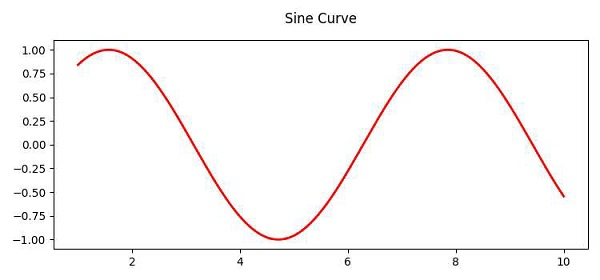# Increase the distance between the title and the plot in Matplotlib

To increase the distance between the title and the plot in matplotlib, we can take the following steps −

• Create point x using numpy.

• Create point y using numpy sin.

• Set the title of the plot. After changing the value y (in argument), we can increase or decrease the distance between the title and the plot.

• Plot x and y points using the plot() method, where color is red and line width is 2.

• Ti display the figure, use the show() method.

## Example

import numpy as np
from matplotlib import pyplot as plt
plt.rcParams["figure.figsize"] = [7.50, 3.50]
plt.rcParams["figure.autolayout"] = True
x = np.linspace(1, 10, 1000)
y = np.sin(x)
ttl = plt.title('Sine Curve', y=1.05)
plt.plot(x, y, c="red", lw=2)
plt.show()

## Output